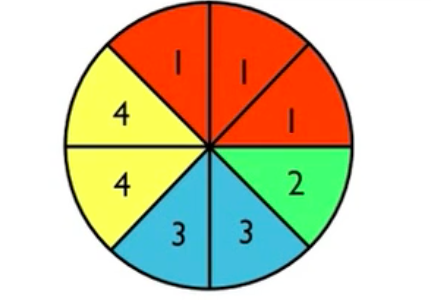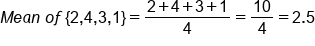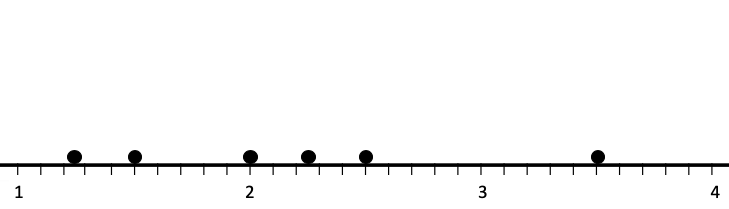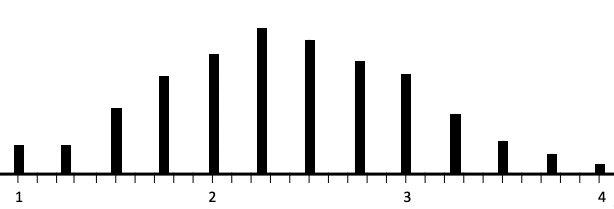+
Introduction to Sampling Distribution
Central Tendency, Variation, and Distributions
0
of 90 possible points

# Introduction to Sampling Distribution

Author: Sophia Tutorial
##### Description:

Identify a sampling distribution for a given scenario.

(more)

Sophia’s self-paced online courses are a great way to save time and money as you earn credits eligible for transfer to many different colleges and universities.*

No credit card required

37 Sophia partners guarantee credit transfer.

299 Institutions have accepted or given pre-approval for credit transfer.

* The American Council on Education's College Credit Recommendation Service (ACE Credit®) has evaluated and recommended college credit for 33 of Sophia’s online courses. Many different colleges and universities consider ACE CREDIT recommendations in determining the applicability to their course and degree programs.

Tutorial
what's covered
This tutorial will explain the sampling distribution of the sample means. Our discussion breaks down as follows:

1. Sampling Distribution

## 1. Sampling Distribution

A sampling distribution of sample means is a distribution that shows the means from all possible samples of a given size. Let’s start with an example of a sampling distribution.

Consider the spinner shown here:Suppose you spin it four times to obtain an average. You get a 2 the first time, a 4 the second time, a 3 the third time, and a 1 the fourth time. The mean is the average of 2, 4, 3, and 1 is:So, your first mean is 2.5.

Sample Mean
S1 = {2, 4, 3, 1} 1 = 2.50

However, your mean won't be 2.5 every time. Suppose you repeat this process five more times to get the following six samples:

Sample Mean
S1 = {2, 4, 3, 1} 1 = 2.50
S2 = {1, 4, 3, 1} 2 = 2.25
S3 = {4, 2, 4, 4} 3 = 3.5
S4 = {2, 2, 3, 1} 4 = 2.00
S5 = {3, 1, 1, 1} 5 = 1.50
S6 = {1, 1, 1, 2} 6 = 1.25

So how can we represent all these distributions?

step by step
Step 1: First, take these sample means and graph them. Draw out an axis. For this one, it should go from 1 to 4 because this set can’t average anything higher than four or lower than a one.
Step 2: Take the average value, for example, the mean of 2.5, and put a dot at 2.5 on the x-axis, much like a dot plot. Do this for all the sample means that you have found.Step 3: You can keep doing this over and over again. Ideally, you would do this hundreds or thousands of times, to show the distribution of all possible samples that could be taken of size four. Once you’ve enumerated every possible sample of size four from this spinner, then the sampling distribution looks like this:On the graph, the lowest number you can get is one, and the highest number you can get is four. On the far right of the graph is the point that represents a spin of 4 fours, {4, 4, 4, 4}. On the far left is the point that represents a spin of 4 ones, {1, 1, 1, 1}. Notice that 4 ones happens more than 4 fours. Why is that? If you take a look at the spinner, you'll see that there are more ones on the spinner than there are fours.

You can also notice that, since there are more ones, this actually pulls the average down a bit. The most frequent average is 2.25, not 2.5, which would be the exact middle between 1 and 4. Therefore, this distribution is skewed slightly to the right because the numbers on the spinner are not evenly distributed.

term to know

Sampling Distribution of Sample Means
A distribution that shows the means from all possible samples of a given size.

summary
A sampling distribution is the distribution of all possible means that you could have for a given size. For example, in a sampling distribution where you graph all the possible means for samples of size 4, you take the sample, calculate the mean, and plot it with a dot. Then you take another sample, calculate the mean again, and plot that, and so forth. It can be a long and tedious process! In large populations, the sampling distribution consists of a very large number of points.

Good luck!

Source: Adapted from Sophia tutorial by Jonathan Osters.

Terms to Know
Sampling Distribution of Sample Means

A distribution that shows the means from all possible samples of a given size.

Rating